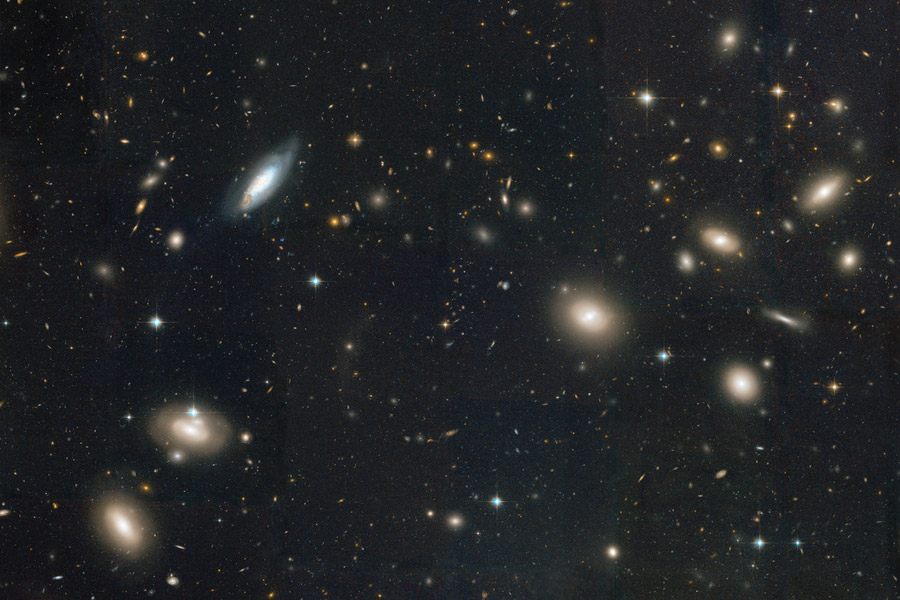# Dark Matter Evidence: Galactic ClustersImage of the Coma Cluster taken by Hubble. Credit: NASA, ESA, Hubble Heritage (STScI/AURA). Per NASA rules, image in public domain.

In the latest entry in my Physics for Non-Physicists series, I will go over some of the evidence for dark matter from measurements of galactic clusters. This post is more of a historical overview of the subject than a review of the most recent literature.

I gave a very brief introduction to dark matter in my previous post on the thermal history of the universe. To recap, dark matter is a form of matter believed to exist that does not interact much with either itself or with the particles of the Standard Model.

As far back as the 1930s (and possibly earlier), the theoretical background and experimental methods of astrophysics had advanced to the point where things like the velocities and luminosities of far-away galaxies could be measured with a reasonable amount of accuracy.

Galactic clusters are a structure containing many galaxies and associated gas in a gravitationally-bound system. In cases where the effects of general relativity can be ignored with little effect (i.e. most cases), gravity follows a Kepler potential: the gravitational potential energy is proportional to inverse distance while the gravitational force is proportional to the square of the inverse distance. For a Kepler potential, it can be shown that the center-of-mass (removing any overall motion) kinetic energy (T, calculated from taking the sum of the kinetic energies of all objects in the cluster) has a particular relationship to the internal gravitational potential energy (U): 2T = -U. This is known as the virial theorem.

Still in the 1930s, it was generally assumed that stars and galaxies follow an approximately constant mass-to-luminosity ratio. That is, when we look at large enough objects, the amount of light has a  known relation to the amount of mass, so by measuring the luminosity, the mass could be estimated. The gravitational potential energy between two objects is -G m1m2/r where G is Newton’s gravitational constant, the m‘s are the masses, and r is the distance between them. From dimensional analysis, the total potential energy of the cluster is$U = - \frac{xGM^2}{R}$

where M is the total mass, R is an approximate radius (size) of the cluster, and x is a constant of order 1 related to the shape of the cluster (spherical, ellipsoidal, etc). The kinetic energy is the sum of 1/2 mv2 (half of mass times center-of-mass velocity squared) for all the objects in the cluster. This can be estimated from a representative sample of galaxies in the cluster, since this can be rewritten as (1/2)MV2 where again M is the total mass and now V2 is the estimated mean squared velocity. The velocity can’t be directly measured because the distances are so incredibly large that motion cannot be detected. Instead, the velocity along the line-of-sight direction can be determined by measuring the light spectrum (amount of light at different wavelengths). Certain spectral features occur at known wavelengths. Motion along the line of sight causes redshift (motion away from us) or blueshift (motion toward us), which are terms for the Doppler effect when applied to light. The sound version of the Doppler effect is something most people have noticed in everyday life: As fast moving objects emitting sound approach and then recede from us, the pitch (frequency/wavelength) of the sound changes. Returning to the topic at hand, by applying the virial theorem, we can see that the mass is given by$M = \frac{RV^2}{Gx}$.

In the early 1930s, an astronomer named Fritz Zwicky was studying the properties of the Coma Cluster (shown at the top of this post). Using the above results, he compared the mass as estimated from the virial theorem to the mass as estimated from the luminosity. The result was surprising: What I’ll call the “virial mass” was much larger than the “luminosity mass:” the galaxies in the cluster seemed to be moving much too fast. Zwicky’s 1937 paper in Ap.J. can be found here. An earlier paper was published in 1933, but it’s not in English so I cannot read it.

Zwicky notes that there are several ways to explain the discrepancy between the estimated masses. One interesting way is that on very large scales (the mass-to-luminosity ratio was for nearby star systems), the gravitational mass is dominated by some form of matter that is not emitting light (i.e. is “dark matter”). This is one of the earliest and most influential proposals for a theory of dark matter. It turns out that Zwicky actually overestimated the discrepancy by a significant amount. More modern values, using a much stronger understanding of astrophysics, still show a discrepancy – our evidence for dark matter has also become much more convincing – but it’s more modest than Zwicky’s, which showed the “virial mass” to be around 100 times the “luminosity mass.”